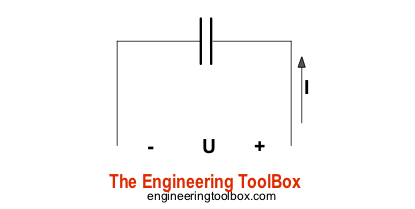Engineering ToolBox - Resources, Tools and Basic Information for Engineering and Design of Technical Applications!

# Capacitors - Stored Energy

## Potential power and energy stored in capacitors.### Capacitor - Energy Stored

The work done in establishing an electric field in a capacitor, and hence the amount of energy stored - can be expressed as

W = 1/2 C U2                     (1)

where

W = energy stored - or work done in establishing the electric field (joules, J)

C = capacitance (farad, F, µF)

U = potential difference (voltage, V)

### Capacitor - Power Generated

Since power is energy dissipated in time - the potential power generated by a capacitor can be expressed as

P = dW / dt                    (2)

where

P = potential power (watts, W)

dt = dissipation time (s)

### Example - Capacitor, energy stored and power generated

The energy stored in a 10 μF capacitor charged to 230 V can be calculated as

W = 1/2 (10 10-6 F) (230 V)2

= 0.26 J

in theory - if this energy is dissipated within 5 μs the potential power generated can be calculated as

P = (0.26 Joules) / (5 10-6 s)

= 52000 W

= 52 kW

Be aware that in any real circuit, discharge starts at a peak value and declines. The energy dissipated is a very rough average power over the discharge pulse.

### Capacitor - Time to Discharge at Constant Power Load

The time to discharge a capacitor at constant power load can be expressed as

dt = 1/2 C (Us2 - Uf2) / P               (3)

where

dt = discharge time (s)

Us = start voltage (V)

Uf = final voltage (V)

## Related Topics

• ### Electrical

Electrical units, amps and electrical wiring, wire gauge and AWG, electrical formulas and motors.
• ### Environment

Climate, meteorology, sun, wind and environmental related engineering resources.

## Related Documents

• ### Capacitors

Capacitors and capacitance - charge and unit of charge.
• ### Capacitors - Parallel and Serial Connections

Parallel and serial connected capacitor circuits.
• ### Energy Storage Density

Energy density - by weight and volume - for some ways to store energy
• ### Salt Hydrates - Melting points and Latent Energy

Melting points and latent energy of salt hydrates.

## Engineering ToolBox - SketchUp Extension - Online 3D modeling!

Add standard and customized parametric components - like flange beams, lumbers, piping, stairs and more - to your Sketchup model with the Engineering ToolBox - SketchUp Extension - enabled for use with older versions of the amazing SketchUp Make and the newer "up to date" SketchUp Pro . Add the Engineering ToolBox extension to your SketchUp Make/Pro from the Extension Warehouse !

We don't collect information from our users. More about

## Citation

• The Engineering ToolBox (2008). Capacitors - Stored Energy. [online] Available at: https://www.engineeringtoolbox.com/capacitors-energy-power-d_1389.html [Accessed Day Month Year].

Modify the access date according your visit.

9.19.12